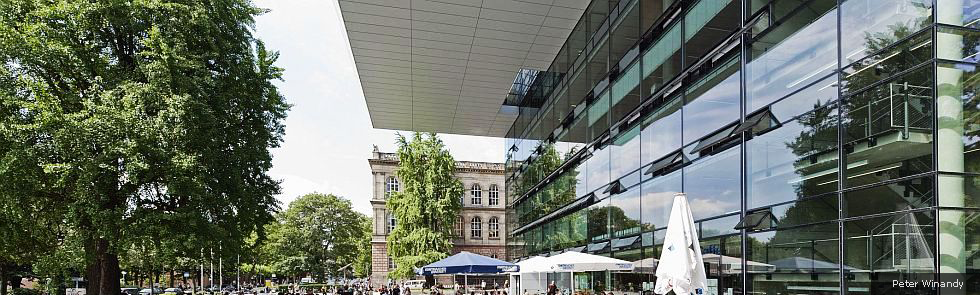Kategorien
Seiten
-## EU Regional School Videos 2020 Part 2

04. August 2020 | von

Course 6 – Prof. Leszek Demkowicz, Ph.D. – The Discontinuous Petrov-Galerkin (DPG) Method (with Optimal Test Functions)

The 3h short course will cover the fundamentals of the DPG method:
1. Three hats of the ideal and practical Petrov-Galerkin method with
optimal test functions.
2. Breaking forms and test spaces.
3. Implementation aspects and examples of computations.
The presentation is based on the chapter in 
 L. Demkowicz, „Lecture Notes on Mathematical Theory of Finite Elements“,
Oden Institute Report 2020/11, May 2020.

Course 8 – Prof. Dr. Felix Krahmer – Structure and Randomness in Data Science

The goal of this short course is to convince you that a smart combination of structure and randomness can be very useful for many data science applications, including sensing and data acquisition, dimension reduction, and computational tasks. Randomness can help, as random parameter choices often lead to good conditioning with high probability due to concentration phenomena, while structure arises either as imposed by applications, or to make the computations more feasible. Here the role of randomness can be twofold. On the one hand, randomness can help establish deterministic properties which are too complex in nature to be understood for any deterministic constructions. For example, a sufficient condition for guaranteed recovery of sparse signals in compressive sensing is the so-called Restricted Isometry Property, that holds when the sensing matrix acts as an approximate isometry on sparse vectors. This property is known to hold for random matrices of embedding dimension  near-linear in the sparsity level with high probability, while no comparable deterministic construction is known to date. This way of thinking can also help in the analysis of stochastic partial differential equations.
In many applications related to computing, in contrast, randomness plays another essential role: A random preprocessing of the data, such as a random projection or a random subsampling, can allow to reduce high dimensional problems to lower dimensional problems that can be solved more efficiently. In most of these scenarios, comparable deterministic constructions are not feasible, as for any realization of the preprocessing operation, the procedure will fail for some data sets. The role of randomness in this context is that it translates a method that works for most instances – which may be useless as the actual data set may be one of the bad instances – into a method that for any data set works with high probability. Structure is important to ensure that the preprocessing remains efficient and its computational complexity does not exceed the one of the task to be performed. Examples of problems where this strategy is of use include nearest neighbour search and principal component analysis.
In the course, we will give an overview of instances of both these paradigms from various application areas and also present some key ideas of the underlying mathematical theory.